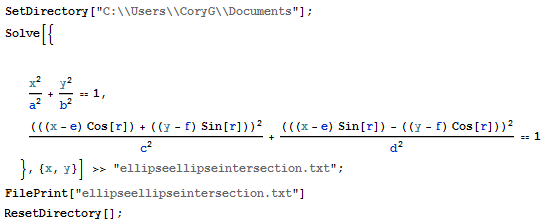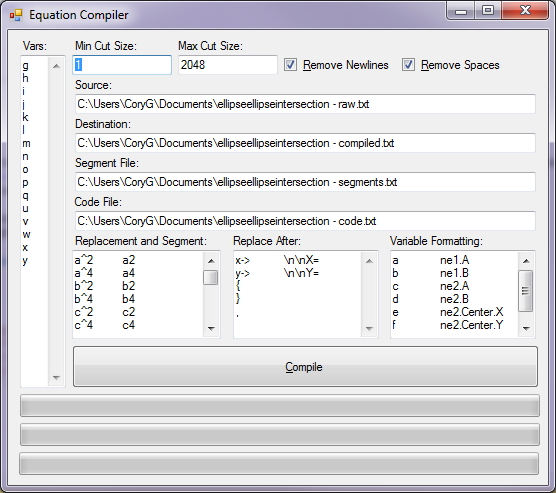# Mathematica Equation Compressor and C# ConverterRecently, I needed to find the intersections of two rotated ellipses with differing center points in c#, it turns out the equations to do this are enormous (113MB directly from Mathematica, 78.2MB after removing spaces and newlines) – so I needed a compressor to shrink the equations down to size (Mathematica’s FullSimplify[] Simplify[] and Reduce[] functions all time out, and pushing the timeout past about a day uses up 16GB of RAM with a ~120GB paging file), and subsequently a way to easily format the equation for insertion into c# (I have a bunch more of these to do, but I’ll use the ellipse-ellipse intersection equations as an example).  The program on this page working in my case to shrink the equations from the initial ~113MB down to 38.7KB – I’m not making the claim it is guaranteed to work – or even that it is safe to run on your machine, but in my case it worked wonderfully.Here is a screenshot of the commands given to Mathematica (the first ellipse is normalized to be centered on [0, 0] and have no tilt, as this is a simple transformation to get to and after about a week my computer produced no results before maxing out it’s 120GB paging file trying to solve for two ellipses with variable center-points and rotations).

I won’t upload the raw output, since it is between 78.2MB and 113MB as described above, but for anyone that found this page looking for the ellipse-ellipse intersections here are links to the compiled equations and the segments of the compiled equations – the c# code is further down.The Equation Compiler

The Vars field on the left controls which variables are used during the compression process (and how many iterations occur).  The Min Cut Size and Max Cut Size fields control the size of cuts made in the equation (in terms of size of each cut, the actual weighting of what to cut is determined by the size*count of each potential cut to maximize size reduction).  Remove Newlines and Remove Spaces act at the start of the process to do just as you would expect of them.  The Source field determines the input file, the next 3 fields determine the various output files.  The Replacement and Segment field determines hard-coded segments (a straightforward find/replace operation, the modifications are included in the segment and code files).  The Replace After field controls a find/replace operation done after all other operations except variable formatting, it takes place in all files (\n is parsed in these parameters as an Environment.Newline string).  The Variable Formatting field controls a find/replace operation that only occurs on the code file (after combining the segment and destination files to form it and doing some other operations like parsing out a^b sections to replace with Math.Pow(a, b) and replacing Sqrt[a] with Math.Sqrt(a), etc) – the trig functions included use a custom class [ie: Angle.GetCos(a), Angle.GetSin(a), etc] – so if you need to convert a trig function you might need to change these in the source yourself.

(source) (output)